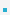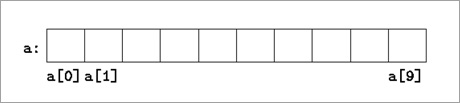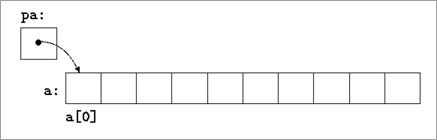3. Pointers and ArraysIn C, there is a strong relationship between pointers and arrays, strong enough that pointers and arrays    should be discussed simultaneously.Primitive arrays implemented in C using pointer to block of contiguous memory.Define an array size of 10, that is a block of 10 consecutive objects named a, a, ..., a.int a, x; int *pa; pa = &a;  // pa contains the address of a pa = a;        // Since the name of an array is a synonym                  // for the location of the initial element x = *pa;      // will copy the contents of a into xThe sizeof() OperatorFor primitive types/variables, size of type in bytes:
 sizeof(char);            // 1 sizeof(double);        // 8 (64-bit OS)For primitive arrays, size of array in bytes:Array length:
 int arr;                // sizeof(arr) == 32 (64-bit OS) long arr ;            // sizeof(arr) == 40 (64-bit OS)Array length
 /* needs to be on one line when implemented */ #define array _length(arr) ＼ ( sizeof(arr) == 0? ＼ 0 : sizeof(arr)/ sizeof((arr)))

 이전페이지 / 4 / 다음페이지